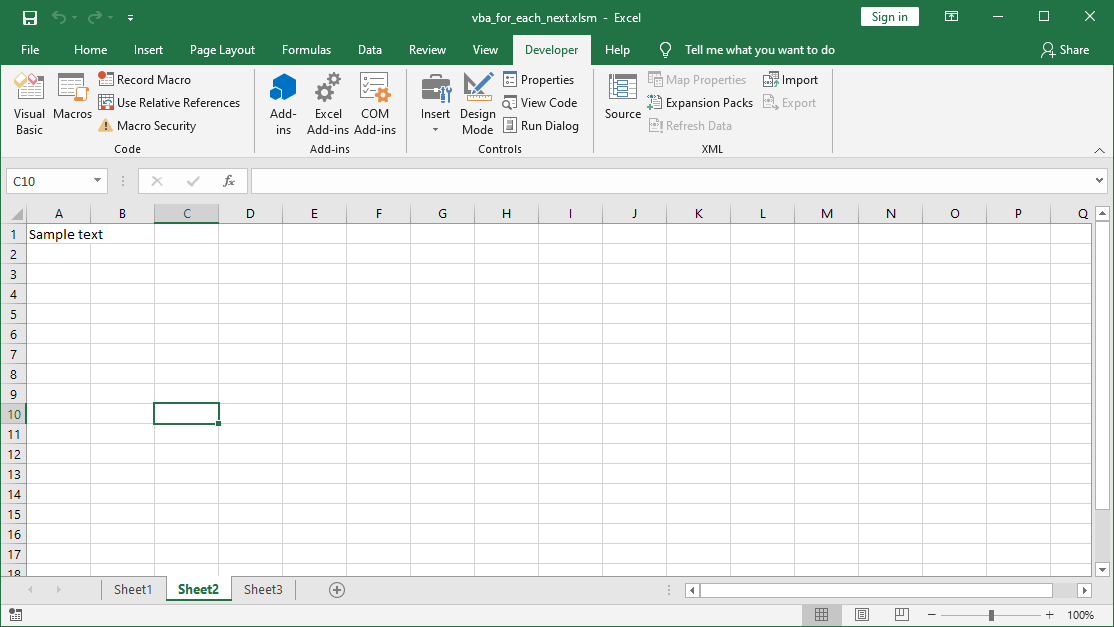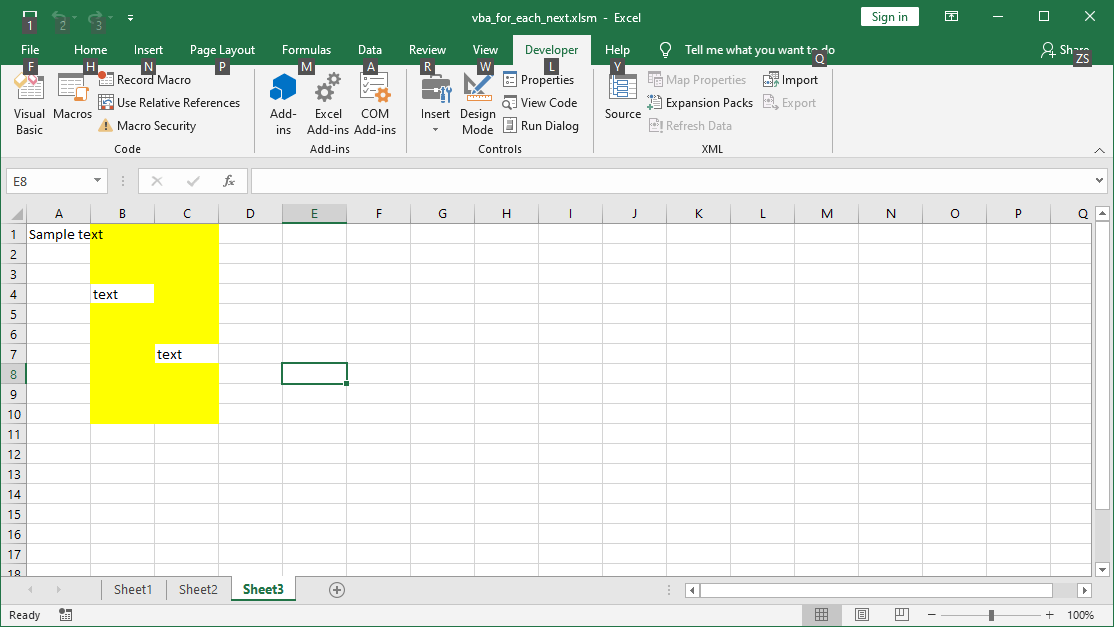# VBA For Each Next Loop

## What is the For Each Next loop in VBA?

In this article you will learn what the For Each Next loop is and how to use it in Excel VBA. The For Each Next Loop is an extension of the VBA For loop. The difference between the two types of loops is that For Each we can apply to a certain group of elements, for example to:

• all cells in the range
• all sheets
• all pivot tables, etc.

The syntax of the VBA For Each Next loop looks as follows:

```For Each element [ As datatype ] In group
[ statements ]
Next [ element ]```
• element – element to which you refer in the group, ie.: every cell, every sheet, etc.
• group – a group of elements eg all sheets, cells in the range, pivot tables, etc.
• statements – commands that will be execute on all elements in a group. For example, colour all cells, hide all sheets, etc.

The VBA for each next loop works on elements. The most commonly used elements in the loop are:

• Worksheet in the Worksheets group  – sheet
• PivotTable in the PivotTables group – pivot tables
• element in the Range group (“X:X”) – cells in the range group

## Examples of VBA For Each Next

Example 1 : Below is the simplest example of the For Each Loop. The example is to enter any text into the A1 cell in any sheet.

```Sub PetlaForEach1()
Dim arkusz As Worksheet

For Each arkusz In ActiveWorkbook.Worksheets
arkusz.Range("A1") = "Sample text"
Next arkusz

End Sub```

Example description: As you can see, the element in the loop is the sheet defined at the beginning of the code as Worksheet. The loop on each sheet inserts the text in cell A1. The group of objects in the loop are the sheets in the active workbook labeled  ActiveWorkbook.Worksheets.Examples of VBA For Each Next. Text in all sheets

Example 2 : Let’s create a For Each Next loop, which searches for empty cells in the B1: c10 cell range. Then colour interior of empty cells with yellow color.

```Sub PetlaForEach2()
For Each element In Range("B1:C10")
If IsEmpty(element.Value) = True Then
element.Interior.ColorIndex = 6
End If
Next
End Sub```

Example description: As you can see, the cell marked as an element in the range is searching for an empty value. If the For Each Next loop found an empty value, it colour the cell in yellow. You can read more about VBA cell formatting in this part of the course: VBA Formatting cells and fonts.VBA For Each Next. Color all empty elements

Some other examples of the VBA For Each Next loop:

• Display information about PivotTables
• Discovering all hidden sheets in VBA
• Refreshing all VBA pivot tables

The article is part of the Excel vba course. The file with the program code: